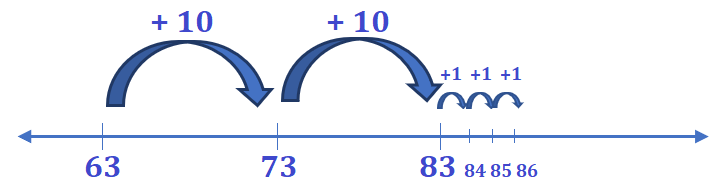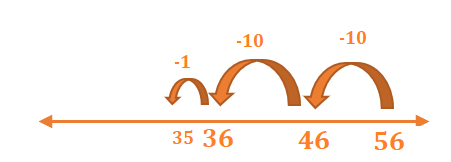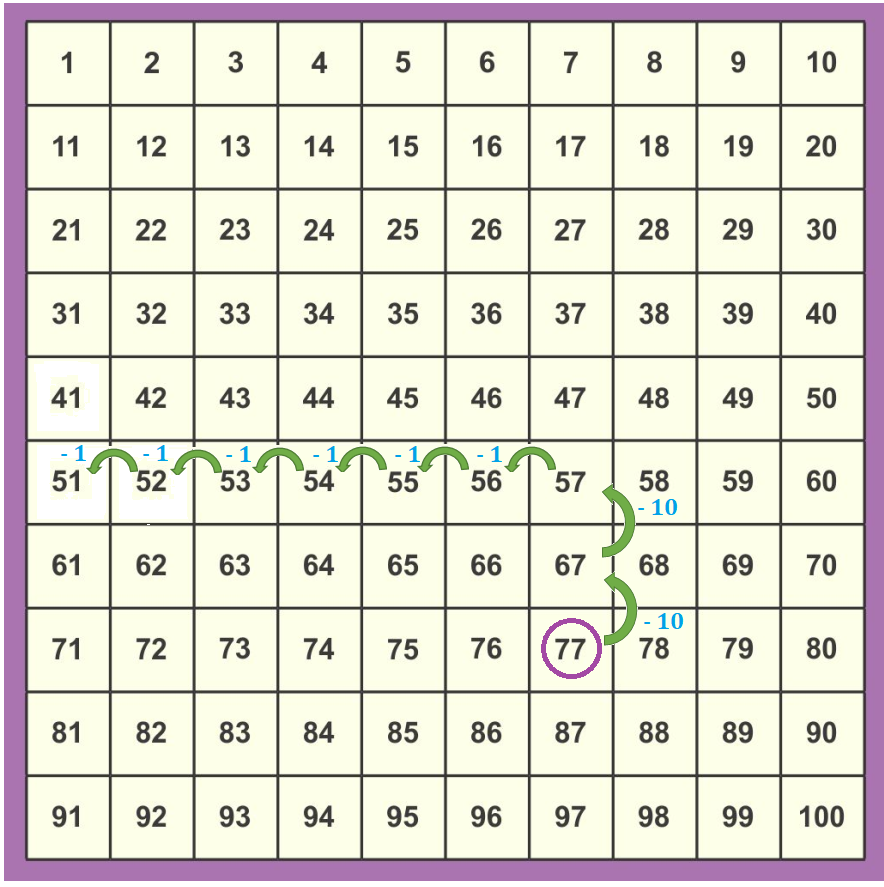# Math > Year 2

## Adding and Subtracting 10s and 1s

What are two-digit numbers?
Two-digit numbers are made up of 2 digits- Tens and Ones.
For example, 34 is made up of 30 and 4.

Adding and Subtracting tens and ones
To add tens and ones, we can use a number line and number square.

Number line method

Example 1: 63 + 23

Step 1: Start from the bigger number.

Step 2: Split the second number.
23 = 10 + 10 + 1 + 1 + 1

Step 3: Count on in tens ones.So, 63 + 23 = 86

Example 2: 56 – 21

Step 1: Start from 56.

Step 2: Split 21 into tens and ones.
21 = 10 + 10 + 1

Step 3: Count back in tens and onesSo, 56 – 21 = 35

Number square method

Example 1: 41 + 34

Step 1: Start from 41.

Step 2: Go three columns downwards as we have 3 tens.

Step 3: Go 4 steps to the right as we have 4 ones.Example 2: 77 – 26

Step 1: Find 77 on a number square.

Step 2: Move two columns upwards as we have 2 tens in 26.

Step 3: Move 6 steps backwards as we have 6 ones in 26.//